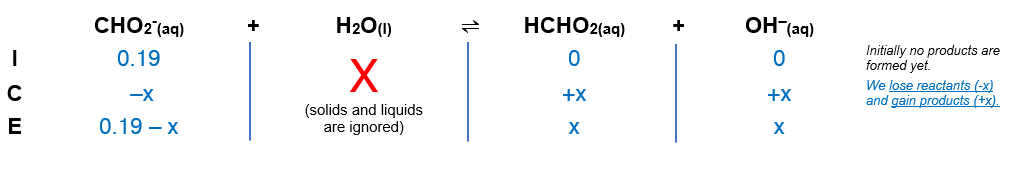# Problem: Determine the pH of each of the following solutions.0.19 M KCHO2 (Ka for HCHO2 is 1.8×10−4)

###### FREE Expert Solution

We’re being asked to calculate the pH of a 0.19 M KCHO2

0.19 M KCHO2 (Ka for HCHO2 is 1.8×10−4)

CHO2- is a weak base that will further react in water to form OH-

KCHO2  (aq) + H2O (l) → OH- (aq) + HCHO2 (aq) + K+ (aq)

From this, we can construct an ICE table for the reaction of CHO2in a solution. Remember that liquids are ignored in the ICE table.92% (242 ratings)###### Problem Details

Determine the pH of each of the following solutions.

0.19 M KCHO2 (Ka for HCHO2 is 1.8×10−4)